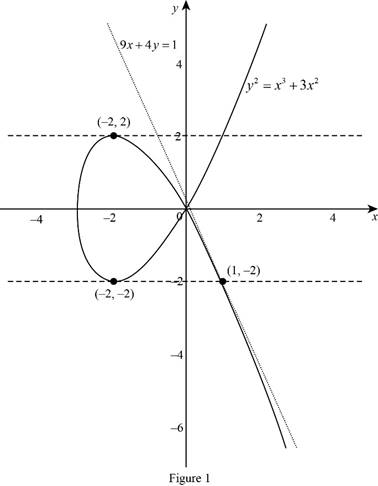# The equation of the tangent line to the given equation at the point.### Single Variable Calculus: Concepts...

4th Edition
James Stewart
Publisher: Cengage Learning
ISBN: 9781337687805### Single Variable Calculus: Concepts...

4th Edition
James Stewart
Publisher: Cengage Learning
ISBN: 9781337687805

#### Solutions

Chapter 3.5, Problem 30E

(a)

To determine

## To find: The equation of the tangent line to the given equation at the point.

Expert Solution

The equation of the tangent line to the equation y2=x3+3x2 at the point (1,2) is 9x+4y=1.

### Explanation of Solution

Given:

The curve with equation y2=x3+3x2.

The point is (1,2).

Derivative rules:

(1) Chain rule: If y=f(u) and u=g(x)  are both differentiable function, then

dydx=dydududx.

(2) Product rule: If y=f(u) and u=g(x)  are both differentiable function, then

ddx(f(x)+g(x))=ddx(f(x))+ddx(g(x)).

Formula used:

The equation of the tangent line at (x1,y1) is, yy1=m(xx1) (1)

Here, m is the slope of the tangent line at (x1,y1) and m=dydx|x=x1,y=y1.

Calculation:

Obtain the equation of tangent line to the given point.

y2=x3+3x2

Differentiate the above equation implicitly with respect to x,

ddx(y2)=ddx(x3+3x2)ddx(y2)=ddx(x3)+3ddx(x2)ddx(y2)=3x2+6x

Apply the chain rule (1) and simplify the terms,

ddy(y2)dydx=3x2+6x2ydydx=3x2+6xdydx=3x2+6x2y

Therefore, the derivative of the equation is dydx=3x2+6x2y.

The slope of the tangent line at (1,2) is computed as follows,

m=dydx|(x1,y1)=(1,2)=3(1)2+6(1)2(2)=94

Thus, the slope of the tangent line at (1,2) is m=94.

Substitute (1,2) for (x1,y1) and m=94 in equation (1),

y+2=94(x1)y+2=9x4+944y+84=9x4+949x+4y=1

Therefore, the equation of the tangent line to the equation y2=x3+3x2 at the point (1,2) is 9x+4y=1.

(b)

To determine

### To find: The points if the curve has horizontal tangent.

Expert Solution

The curve has horizontal tangent at (2,2) and (2,2).

### Explanation of Solution

Given:

The curve with equation y2=x3+3x2.

The derivative of the equation dydx=3x2+6x2y.

Calculation:

Obtain the point if the curve has horizontal tangent.

Note that, the curve horizontal tangent if dydx=0.

Suppose that dydx=0,

3x2+6x2y=03x2+6x=0x(3x+6)=0x=0  or  x=2

Rewrite the given equation as follows,

y=x3+3x2

Substitute x=0 in the above equation,

y=03+3(0)2=0

The derivative of the equation does not exist at (0,0).

Substitute  x=2 in y=x3+3x2,

y=(2)3+3(2)2=8+12=4=±2

Therefore, the curve has horizontal tangent at (2,2) and (2,2).

(c)

To determine

Expert Solution

### Explanation of Solution

Graph:

Using online graphic calculator to draw the curve and the tangent line as shown below in Figure 1,From Figure 1, it is observed that the line 9x+4y=1 touch the curve y2=x3+3x2 at (1,2). That is, the tangent line 9x+4y=1 to the curve at (1,2) and the curve has horizontal tangent at (2,2) and (2,2).

### Have a homework question?

Subscribe to bartleby learn! Ask subject matter experts 30 homework questions each month. Plus, you’ll have access to millions of step-by-step textbook answers!